Next Article in Journal
Construction Industrialization in China: Current Profile and the Prediction
Previous Article in Journal
A Review on Natural Ventilation-enabling Façade Noise Control Devices for Congested High-Rise Cities
Article

# Voltage-Mode and Current-Mode Resistorless Third-Order Quadrature Oscillator

1
Department of Electronic Engineering, Ming Chi University of Technology, New Taipei 24301, Taiwan
2
Department of Electronic Engineering, National Taipei University of Technology, Taipei 10608, Taiwan
*
Author to whom correspondence should be addressed.
Appl. Sci. 2017, 7(2), 179; https://doi.org/10.3390/app7020179
Received: 31 December 2016 / Accepted: 6 February 2017 / Published: 13 February 2017

## Abstract

This paper presents the realization of a resistorless third-order quadrature oscillator using two multiple-output current controlled current conveyor transconductance amplifiers (MO-CCCCTAs) and three grounded capacitors. The proposed circuit provides two quadrature voltage outputs, two high-impedance quadrature current outputs, and one high-impedance amplitude-controllable current output. The proposed oscillator satisfies the industrial requirement for amplifier and modulation signals and phase shift keying signals, when the input bias current of the second MO-CCCCTA is used as a modulating signal. Its oscillation condition and oscillation frequency can be adjusted independently by two different bias currents of MO-CCCCTAs. The structure of resistorless and only grounded capacitors suggests that the proposed oscillator can be easily implemented in an integrated circuit.
Keywords:

## 1. Introduction

A quadrature sinusoidal oscillator is a very important active element for communication, instrumentation, and measurement systems, and can be employed in a quadrature mixer and a single-sideband modulator of telecommunications [1,2]. In communication systems, a sinusoidal oscillator can generate an amplitude-controllable sinusoidal signal, which can be used to apply the carrier signal for amplitude modulation (AM) and amplitude shift keying (ASK) signal systems . Recently, current conveyors are receiving considerable attention due to their potential advantages, such as inherently wide bandwidth, high slew-rate, good linearity, wide dynamic range, simple circuitry, and low power consumption . This interesting active element, based on second-generation current conveyor transconductance amplifiers (CCIITA) , has been introduced to provide new possibilities in the realization of a class of analog signal processing circuits. This CCIITA can provide flexibility in operating both current and voltage modes, and can enable a variety of circuit designs and provides electronic tuning ability through its transconductance gain, gm. However, the CCIITA cannot control the parasitic resistance, RX, at X port. Hence, when the CCIITA is used in some circuits, it must unavoidably require some external passive components, especially with the resistors. In contrast, the introduced current-controlled current conveyor transconductance amplifier (CCCCTA) [5,6] has two electronically adjustable ports, whereas the CCIITA has only one electronically adjustable port. The parasitic resistance, RX, at current input port of the CCCCTA can be adjusted by an input bias current, which does not require a resistor in practical applications. Intuitively, the CCCCTA is a versatile active building block, which provides the possibility of utilizing its transconductance gain, gm, and its equivalent input resistance, namely parasitic resistance, RX, to create a resistorless oscillator scheme. The multiple current output terminals of the CCCCTA can be easily obtained by adding additional current mirrors at these output terminals.
Electronically tunable active components are receiving more attention as traditional electronic components may have deviations in fine-tuning the tolerances of the electronic components . The electronically tunable active components of the previous second-order oscillators include second generation current controlled conveyors (CCCIIs) [8,9]; operational transconductance amplifiers (OTAs) ; current differencing buffered amplifiers (CDBAs) [11,12]; the CCIITA ; current differencing transconductance amplifiers (CDTAs) [14,15,16,17,18,19]; multiple-output current controlled current conveyor transconductance amplifiers (MO-CCCCTAs) ; and voltage differencing transconductance amplifiers (VDTAs) . These circuits were used to design the second-order quadrature current outputs, quadrature voltage outputs, or both quadrature current and voltage outputs. In addition to all of the above second-order quadrature oscillators, several third-order quadrature oscillators have also been proposed [22,23,24,25,26,27,28,29,30,31,32].
The third-order oscillators have higher accuracy, higher quality factor, and lower harmonic distortion than the second-order oscillators [22,23]. However, the third-order oscillator in Figure 7 of Reference  uses three OTAs and three grounded capacitors. The third-order oscillator in Reference  uses four multiple-output second-generation current-controlled conveyors (MO-CCCIIs) and three grounded capacitors. The third-order oscillator in Figure 1 of Reference  uses three second-generation current conveyors (CCIIs), five grounded resistors and three capacitors. The third-order oscillator in Reference  uses two MO-CCCCTAs and three grounded capacitors. The third-order oscillator in Figure 3 of Reference  uses three voltage controlled differential voltage current conveyors (VC-DVCCs) and three grounded capacitors. The third-order oscillator in Reference  uses three MO-CCCIIs and three grounded capacitors. The third-order oscillator in Reference  uses three multiple-output current differencing transconductance amplifiers (MO-CDTAs) and three grounded capacitors. Although the third-order oscillator in Reference  uses two multiple-output second-generation current conveyors (MO-CCIIs), three resistors, and three grounded capacitors, one of the resistors is a floating connection. The third-order oscillator in Reference  uses three multiple-output differential voltage current conveyors (MO-DVCCs), three resistors, and three grounded capacitors; however, one of the resistors is also a floating connection. The oscillator in Figure 2 of Reference  uses three operational transresistance amplifiers (OTRAs), four resistors and three capacitors; however, not all of the resistors and capacitors are grounded.
In 2015, two new third-order quadrature oscillators were proposed , which employed two multiple-output differential voltage current conveyor transconductance amplifiers (MO-DVCCTAs), one/two resistors, and three grounded capacitors. However, these circuits [22,23,24,25,26,27,28,29,30,31,32] suffered from one or more of the following drawbacks: (i) the inclusion of three/four active components [22,23,24,26,27,28,30,31]; (ii) the lack of both a voltage-mode and current-mode quadrature oscillator in one circuit topology [22,23,24,25,31]; (iii) the lack of a resistorless oscillator [24,29,30,31,32]; (iv) the lack of an independently electronically tunable for the oscillation condition and frequency [22,24,30,32]; (v) the lack of amplitude-controllable for output current signal [22,23,24,25,26,27,28,29,30,31,32]; and (vi) the lack of quadrature voltage outputs, quadrature current outputs, and a high-impedance amplitude-controllable current output in one circuit topology [22,23,24,25,26,27,28,29,30,31,32], especially, the electronically controllable amplitude of the sinusoidal current output signal that can be easily used in AM/ASK systems.
In this paper, a new approach to realize third-order quadrature oscillators using a lossy integrator and two lossless integrators is proposed. The proposed third-order quadrature resistorless oscillator employs two MO-CCCCTAs and three grounded capacitors. The proposed third-order quadrature oscillator has the following seven advantages simultaneously: (i) it contains only two active components and three grounded capacitors; (ii) it has both voltage-mode and current-mode third-order sinusoidal quadrature oscillator in the same topology; (iii) it achieves quadrature voltage outputs, quadrature current outputs, and a high-impedance amplitude-controllable current output in one circuit topology; (iv) it has independent electronically tunable characteristics for the condition of oscillation (CO) and the frequency of oscillation (FO); (v) it provides a high-impedance current output of adjustable magnitude, without effecting the CO and FO and is suitable for AM/ASK modulations; (vi) it uses only grounded capacitors; and (vii) it holds low active and passive sensitivity performances. Table 1 and Table 2 compare the main features of the proposed circuit with previous third-order sinusoidal oscillators [22,23,24,25,26,27,28,29,30,31,32].

## 2. Proposed Method

The proposed realization of a third-order quadrature oscillator is shown in Figure 1, and consists of a current-mode lossy integrator and two current-mode lossless integrators. The system can be analyzed as:
$I 2 I 1 = − a s + a , I 3 I 2 = b s , I 1 I 4 = c s$
$I 4 = I 2 + I 3 = ( 1 + b s ) I 2$
Equation (1) shows the functions of one current-mode inverting lossy integrator and two current-mode non-inverting lossless integrators in the functional block diagram. Substitute Equation (1) into Equation (2), and obtain the relationship between I1 and I4 currents as:
$I 1 I 4 = I 1 ( 1 + b s ) I 2 = c s$
Rearrange Equation (3), and yield the transfer function between I1 and I2 currents as follows:
$I 1 I 2 = ( 1 + b s ) ( c s ) = ( s + b s ) ( c s )$
Then, the characteristic equation of the functional block diagram in Figure 1 can be expressed as:
$s 3 + a s 2 + a c s + a b c = 0$
From Equation (5), the CO and the FO are obtained as follows:
CO: a = b

## 3. Circuit Descriptions

The CCCCTA is an active building block, which is useful in simplifying the circuit design. The CCCCTA device integrates a CCCII and an OTA into a versatile component to facilitate the implementation of analog signal-processing circuits. The CCCCTA does not need a resistor in practical applications, as its transconductance gain, gm, and parasitic resistance, RX, are electronic adjustable. Figure 2 shows the symbol of a multiple-output CCCCTA (MO-CCCCTA) . The MO-CCCCTA properties can be expressed as VX = VY + IXRX, IZ = IZC1 = IZC2 = IX and IO1 = IO2 = gmVZ, where RX is the parasitic resistance of the X-terminal, and gm is the transconductance gain of the MO-CCCCTA. The bias currents, IS and IB, of MO-CCCCTA can control the parasitic resistance, RX, and the transconductance gain, gm, respectively [33,34]. Figure 3 shows the proposed schematic of the resistorless third-order quadrature oscillator, which consists of two MO-CCCCTAs and three grounded capacitors. The only grounded capacitors without a resistor structure in the proposed circuit are easily integrated into a single chip. In Figure 3, the transfer function, B/A, realizes a current-mode lossy integrator, and the transfer functions, C/B and D/C, realize two current-mode lossless integrators.
Let D = A, and the close-loop circuit analysis provides the following system characteristic equation.
$s 3 C 1 C 2 C 3 R X 1 R X 2 + s 2 C 2 C 3 R X 2 + s C 2 + g m 1 = 0$
and the CO and FO can be obtained as:
As indicated by Equations (9) and (10), the CO can be adjusted by RX1 without disturbing the FO, and the FO can be controlled by RX2 without affecting the CO. In other words, the CO and FO are controlled independently by the bias currents, IS1 and IS2, respectively. As the parasitic resistances, RX1 and RX2, of the MO-CCCCTA can be electronically controlled by IS1 and IS2, respectively, both the CO and FO are independently and electronically controllable. In a steady state, the two voltage outputs and two current outputs in Figure 3 are:
where $k 1 = ω o C 2 R X 1$, and $k 2 = ω o C 2 g m 1$.
Equation (11) shows that the proposed circuit can obtain the quadrature voltage outputs and the quadrature current outputs simultaneously. The magnitude ratios of the quadrature voltage outputs and the quadrature current outputs are the function of the operating frequency. Thus, the value of RX2 will affect the oscillation frequency and the magnitude ratios of the generated quadrature signals. However, this problem can be solved by using C1 = C2 = C3, RX1 = RX2, and letting the product gm1RX1 = 1. Subsequently, the amplitude of voltages and currents in Equation (11) are equal and ensure k1 = k2 = 1.
The output current, Io3, can be given as follows:
$I o 3 = g m 2 V o 1$
where the gm2 of the second MO-CCCCTA can be independently tuned by the bias current, IB2. This means that the gm2-value can be tuned by IB2, without disturbing the CO and FO. Thus, if a modulating signal is applied to IB2, then the AM/ASK signals can be obtained from Io3.
From Equation (11), the magnitude of Vo2 and Vo1 or Io2 and Io1 is dependent on the factor k1 or k2. Therefore, the mechanism of amplitude limitation will depend on the factors k1 and k2. For complementary metal oxide semiconductor (CMOS) implementation of CCCCTA , the RX and gm are written as:
where $k R X = μ n C O X ( W L ) 1 , 2 = μ p C O X ( W L ) 3 , 4$, $k g m = μ n C O X ( W L ) 20 , 21$. RX is the parasitic resistance of the X-terminal and gm is the transconductance gain of the CCCCTA. Here k is the physical transconductance parameter of the MOS transistor. IS and IB are the bias current used to control the parasitic resistance and transconductance gain, respectively. In other words, the variation of CCCCTA bias current range will limit the stability of the output amplitude.

## 4. Phase Noise and Phase Error Analysis

The phase noise, which are the important performance parameters of an oscillator, can be calculated by the method outlined in Reference  and can be represented in the block diagram of Figure 4. The spectral density of the phase noise is defined in Reference .
$| Y X [ j ( ω o + Δ ω ] | 2 = 1 ( Δ ω ) 2 | d H d ω | 2$
where ωo is oscillation frequency and ω = ωo + Δω is carrier frequency approximation.
If $H ( j ω ) = A ( ω ) exp [ j φ ( ω ) ]$, then:
$d H d ω = ( d A d ω + j A d φ d ω ) exp [ j φ ( ω ) ]$
Substitute Equation (15) into Equation (14) and get:
The phase noise of the proposed circuit can be obtained by Equations (14)–(16).
Quadrature oscillators are widely used in communication systems due to its in-phase and quadrature phase signals, which deviate from 90°. Therefore, the quadrature phase accuracy between the two output signals should be reasonable. Generally, the in-phase and quadrature phase expressions of the quadrature oscillator signals can be written as:
$V I ( t ) = V m ( I ) cos ( ω t + θ ( I ) )$
$V Q ( t ) = V m ( Q ) cos ( ω t + θ ( Q ) )$
$θ ( Q ) − θ ( I ) = π 2 + ϕ$
where ω is the oscillation frequency and ϕ is the phase deviation from the quadrature signal. VI(t) is the in-phase signal and VQ(t) is the quadrature phase signal. The phase error of the proposed circuit can be obtained by Equations (17)–(19).

## 5. Non-Ideal Discussion

The terminal voltage and current relationships of the non-ideal ith MO-CCCCTA can be rewritten as VXi = βiVYi + IXiRXi, IZi = αiIX, IZC1i = ηiIXi, IO1i = γigmiVZi and IO2i = λigmiVZi for i = 1, 2, where βi, αi. γi, ηi and λi represent the tracking errors of the MO-CCCCTA voltage and current, respectively . The characteristic equation of the proposed circuit in Figure 3 can be rewritten as:
$s 3 C 1 C 2 C 3 R X 1 R X 2 + s 2 C 2 C 3 R X 2 + α 2 β 2 η 1 s C 2 + α 1 α 2 β 2 γ 1 g m 1 = 0$
In this case, the CO and FO of the non-ideal oscillator can be expressed as:
The active and passive sensitivities of the non-ideal oscillator parameters are shown as:
$S α 1 , α 2 , β 2 , γ 1 ω 0 = S g m 1 ω 0 = − S R X 2 , C 2 , C 3 ω 0 = 1 2$
From Equation (23), the active and passive sensitivities are equal to 0.5, which satisfy the sensitivity performance of the circuit.
The parasitic terminal impedances of the ith non-ideal MO-CCCCTA are (RYi//CYi) of terminal Yi, (RZi//CZi) of terminal Zi, (RZC1i//CZC1i) of terminal ZC1i, (RZC2i//CZC2i) of terminal ZC2i, (RO1i//CO1i) of terminal O1i, and (RO2i//CO2i) of terminal O2i [20,33]. The X, Z, and O1 of the first MO-CCCCTA terminals connect to the external C1, C2 and C3 capacitors in parallel, respectively, as shown in Figure 3. If C1 >> CZ2, C2 >> CZ1 and C3 >> (CO11 + CY2 + CZC11), the effects of the parasitic capacitances, CZ2, CZ1 and CO11, can be ignored. Hence, to minimize the effects of the MO-CCCCTA parasitic terminal impedances, the external capacitor values should be restricted by
$1 s ( C 1 + C Z 2 ) < < R Z 2$
$1 s ( C 2 + C Z 1 ) < < R Z 1$
$1 s ( C 3 + C O 11 + C Y 2 + C Z C 11 ) < < R O 11 / / R Y 2 / / R Z C 11$

## 6. Simulation Results

To verify the theoretical analysis of the proposed circuit, an H-Spice simulation with Taiwan Semiconductor Manufacturing Company (TSMC) 0.18 µm process was performed. Figure 5 shows the CMOS schematic of a MO-CCCCTA , where the multiple outputs are multiple current mirrors. The aspect ratios (W/L) of the MOS transistors of Figure 5 are given in Table 3. The supply voltages are VDD = −VSS = 0.9 V. In order to get the fo ≅ 2.12 MHz oscillation frequency of the sinusoidal output waveforms, the values of the active and passive components have been chosen as gm1 = gm2 = 133.33 µA/V (IB = 42.9 µA); RX1 = RX2 = 7.5 kΩ (IS = 3.96 µA); C1 = 10.1 pF; and C2 = C3 = 10 pF. The variation of the transconductance value changes IB from 1.5 μA to 210 μA as depicted in Figure 6. When the bias current is larger than 160 μA, the transconductance gain is decreased as the transistors (M20, M21) enter the linear region from a saturation region. The maximum transconductance gain is approximately 256 µA/V. In other words, when the bias current is larger than 160 μA, the internal construction transistors of the MO-CCCCTA operates in the linear region, which will result in the distortion of the output swing. The steady state output waveforms of the quadrature voltages are shown in Figure 7 and the quadrature currents are shown in Figure 8. The oscillation frequency, fo, of the simulation results is equal to 2.11 MHz, which is consistent with the theoretical analysis. The total harmonic distortion (THD) analysis of the Vo1, Vo2, Io1 and Io2 are summarized in Table 4, Table 5, Table 6 and Table 7, respectively. Furthermore, the output current, Io3, can be used as an AM or ASK signal generator, when the amplitude of the input bias current, IB2, is applied to a sinusoidal signal, a triangular signal, or a pulse signal. Thus, the proposed oscillator can generate either AM or ASK signals without additional elements. Figure 9 and Figure 10 show the simulated results of the proposed circuit serving as an AM signal generator, when the input bias current, IB2, is applied to a sinusoidal signal or a triangular signal with a 200-kHz frequency. Figure 11 shows the simulated results of the proposed circuit serving as an ASK signal generator when the input bias current, IB2, is applied to a pulse signal with a 200-kHz frequency.
The MO-CCCCTA is not an off-the-shelf component but can be built by commercially available integrated circuits (ICs). For testing the proposed oscillator, Figure 12 shows an equivalent method similar to Figure 3 by using ready-to-use ICs, which include the operational amplifiers of AD844s and the operational transconductance amplifiers of MAX435 and CA3080. The DC power supply voltages are ±5 V. The gm-value of the MAX435 is equal to 4/Rg, where Rg is an external resistor . In general, the applicability of such current-feedback operational amplifier (CFOA)-based oscillators is usually limited to a few hundred kilohertz [37,38]. Figure 13 shows the experimental result of the frequency range of a commercially available AD844 with ±5 V DC supply. In Figure 13, the values of the resistors were chosen as R1 = R2 = 1 kΩ (5 kΩ, 10 kΩ), RL = 500 Ω, CL = 10 pF, and the input power was 0 dBm. Figure 14 is the measured result of a spectrum analyzer, which shows that the measured result of the frequency range of AD844 was limited to 2 MHz. The passive component values of the circuit in Figure 12 were set as gm1 = 1.428 mS (i.e., Rg = 2.8 kΩ), RX1 = 0.73 kΩ, RX2 = 0.7 kΩ, R = 1 kΩ and C1 = C2 = C3 = 750 pF, where RX1 was designed to be larger than the theoretical value to ensure that the oscillator will start, before the centre frequency was obtained as fo = 303.15 kHz. The oscilloscope output waveforms, Vo1 and Vo2, of the proposed oscillator are shown in Figure 15, and the XY plot of Vo1 and Vo2 output voltages are shown in Figure 16. The experimental oscillation frequency in Figure 15 is 295.2 kHz, which is close to the theoretical value of 303.15 kHz with a 2.62% error rate. Figure 17, Figure 18 and Figure 19 show the AM and the ASK signal outputs of the quadrature oscillator, where the modulation signal, IB2, applied a sinusoidal signal, a triangular signal, or a pulse signal with a 30-kHz frequency. The experimental results are close to the theoretical analysis of Equation (12).
The frequency spectrum of the oscillator output voltage, Vo1, is shown in Figure 20. The measured oscillation frequency was 296.68 kHz, which is close to the theoretical value of 303.15 kHz with a 2.13% error rate. The THD, including the first harmonic through to the ninth harmonic components, is approximately 2.35%. The experimental results are consistent with the theoretical values. For oscillators, noise is a major concern where even a small noise in an oscillator will cause dramatic changes in its frequency spectrum and timing properties. Figure 21 shows the phase noise using the Agilent phase noise measurement solution. The phase noise of the proposed oscillator is less than −88.2 dBc/Hz at 10 kHz offset. Figure 22 shows the root mean square (RMS) jitter of the proposed oscillator, which is 6 ns at an operating frequency of 295.2 kHz.

## 7. Conclusions

A new resistorless third-order electronically tunable quadrature oscillator was proposed in this paper, which uses two MO-CCCCTAs and three grounded capacitors. The proposed oscillator provided two quadrature voltage output signals with a 90o phase difference; two high-impedance quadrature current output signals with a 90o phase difference; and one high-impedance current output signal with an electronically controlled amplitude of the sinusoidal signal, all simultaneously. The proposed oscillator can generate either AM or ASK signals for communication systems. Furthermore, the oscillation condition and oscillation frequency are independently adjustable by two different input bias currents of the MO-CCCCTAs. Since the proposed oscillator does not require external resistors and uses only grounded capacitors, it is suitable for integrated circuit implementation. The simulation and experimental results validate the feasibility of the proposed theory.

## Author Contributions

Hua-Pin Chen, Yuh-Shyan Hwang and Yi-Tsen Ku contributed equally in the presented research work.

## Conflict of Interest

The authors declare no conflicts of interest.

## References

1. Holzel, R. A simple wide-band sine wave quadrature oscillator. IEEE Trans. Instrum. Meas. 1993, 43, 758–760. [Google Scholar] [CrossRef]
2. Senani, R.; Bhaskar, D.R.; Singh, V.K.; Sharma, R.K. Sinusoidal Oscillators and Waveform Generators Using Modern Electronic Circuit Building Blocks; Springer: Cham, Switzerland, 2016. [Google Scholar]
3. Siripruchyanun, M.; Jaikla, W. Cascadable current-mode biquad filter and quadrature oscillator using DO-CCCIIs and OTA. Circuits Syst. Signal Process. 2009, 28, 99–110. [Google Scholar] [CrossRef]
4. Bhaskar, D.R.; Sharma, V.K.; Monis, M.; Rizvi, S.M.I. New current-mode universal biquad filter. Microelectron. J. 1999, 30, 837–839. [Google Scholar]
5. Biolek, D.; Senani, R.; Biolkova, V.; Kolka, Z. Active Elements for Analog Signal Processing: Classification, Review, and New Proposals. Radioengineering 2008, 17, 15–32. [Google Scholar]
6. Siripruchyanun, M.; Jaikla, W. Current controlled current conveyor transconductance amplifier (CCCCTA): A building block for analog signal processing. Electr. Eng. 2008, 90, 443–453. [Google Scholar] [CrossRef]
7. Prommee, P.; Khateb, F. High-performance current-controlled CDCCC and its applications. Indian J. Pure Appl. Phys. 2014, 52, 708–716. [Google Scholar]
8. Horng, J.W. A sinusoidal oscillator using current controlled current conveyors. Int. J. Electron. 2001, 88, 659–664. [Google Scholar] [CrossRef]
9. Lahiri, A. Deriving (MO)(I)CCCII based second-order sinusoidal oscillators. Radioengineering 2011, 20, 349–353. [Google Scholar]
10. Bhaskar, D.R.; Abdalla, K.K.; Senani, R. Electronically-controlled current-mode second order sinusoidal oscillators using MO-OTAs and grounded capacitors. Circuits Syst. 2011, 2, 65–73. [Google Scholar] [CrossRef]
11. Horng, J.W. Current differencing buffered amplifiers based single resistance controlled quadrature oscillator employing grounded capacitors. IEICE Trans. Fundam. Electron. Commun. Comput. Sci. 2002, 8, 1416–1419. [Google Scholar]
12. Tangsrirat, W.; Pukkalanun, T.; Surakampontorn, W. CDBA-based universal biquad filter and quadrature oscillator. Act. Passive Electron. Compon. 2008, 2008, 247171. [Google Scholar] [CrossRef]
13. Lahiri, A. Explicit-current-output quadrature oscillator using second-generation current conveyor transconductance amplifier. Radioengineering 2009, 18, 522–526. [Google Scholar]
14. Keskin, A.U.; Biolek, D. Current mode quadrature oscillator using current differencing transconductance amplifiers (CDTA). IEE Proc. Circuits Devices Syst. 2006, 153, 214–218. [Google Scholar] [CrossRef]
15. Tangsrirat, W. Current differencing transconductance amplifier-based current-mode four-phase quadrature oscillator. Indian J. Eng. Mater. Sci. 2007, 14, 289–294. [Google Scholar]
16. Li, Y. Electronically tunable current-mode quadrature oscillator using single MCDTA. Radioengineering 2010, 19, 667–671. [Google Scholar]
17. Biolek, D.; Keskin, A.U.; Biolkova, V. Grounded capacitor current mode single resistance-controlled oscillator using single modified current differencing transconductance amplifier. IEE Proc. Circuits Devices Syst. 2010, 4, 496–502. [Google Scholar] [CrossRef]
18. Kumngern, M.; Lamun, P.; Dejhan, K. Current-mode quadrature oscillator using current differencing transconductance amplifiers. Int. J. Electron. 2012, 99, 971–986. [Google Scholar] [CrossRef]
19. Jaikla, W.; Lahiri, A. Resistor-less current-mode four-phase quadrature oscillator using CCCDTAs and grounded capacitors. AEÜ–Int. J. Electron. Commun. 2012, 66, 214–218. [Google Scholar] [CrossRef]
20. Sa-Ngiamvibool, W.; Jantakun, A. Quadrature oscillator using CCCCTAs and grounded capacitors with amplitude controllability. Int. J. Electron. 2014, 101, 1737–1758. [Google Scholar] [CrossRef]
21. Prasad, D.; Srivastava, M.; Bhaskar, D.R. Electronically controllable fully-uncoupled explicit current-mode quadrature oscillator using VDTAs and grounded capacitors. Circuits Syst. 2013, 4, 169–172. [Google Scholar] [CrossRef]
22. Prommee, P.; Dejhan, K. An integrable electronic controlled sinusoidal oscillator using CMOS operational transconductance amplifier. Int. J. Electron. 2002, 89, 365–379. [Google Scholar] [CrossRef]
23. Maheshwari, S.; Khan, I.A. Current controlled third order quadrature oscillator. IEE Proc. Circuits Devices Syst. 2005, 152, 605–607. [Google Scholar] [CrossRef]
24. Horng, J.W.; Hou, C.L.; Chang, C.M.; Chung, W.Y.; Tang, H.W.; Wen, Y.H. Quadrature oscillators using CCIIs. Int. J. Electron. 2005, 92, 21–31. [Google Scholar] [CrossRef]
25. Lawanwisut, S.; Siripruchyanun, M. High output-impedance current-mode third-order quadrature oscillator based on CCCCTAs. In Proceedings of the IEEE Region 10 Conference (TENCON ’09), Singapore, 23–26 November 2009; pp. 1–4.
26. Maheshwari, S. Quadrature oscillator using grounded components with current and voltage outputs. IET Circuits Devices Syst. 2009, 3, 153–160. [Google Scholar] [CrossRef]
27. Maheshwari, S. Current-mode third-order quadrature oscillator. IET Circuits Devices Syst. 2010, 4, 188–195. [Google Scholar] [CrossRef]
28. Horng, J.W.; Lee, H.; Wu, J.Y. Electronically tunable third-order quadrature oscillator using CDTAs. Radioengineering 2010, 19, 326–330. [Google Scholar]
29. Horng, J.W. Current/voltage-mode third order quadrature oscillator employing two multiple outputs CCIIs and grounded capacitors. Indian J. Pure Appl. Phys. 2011, 49, 494–498. [Google Scholar]
30. Chaturvedi, B.; Maheshwari, S. Third-order quadrature oscillator circuit with current and voltage outputs. ISRN Electron. 2013, 2013, 385062. [Google Scholar] [CrossRef]
31. Pandey, R.; Pandey, N.; Komanapalli, G.; Anurag, R. OTRA based voltage mode third order quadrature oscillator. ISRN Electron. 2014, 2014, 126471. [Google Scholar] [CrossRef]
32. Pandey, N. Approach for third order quadrature oscillator realization. IET Circuits Devices Syst. 2015, 9, 161–171. [Google Scholar] [CrossRef]
33. Maheshwari, S.; Singh, S.V.; Chauhan, D.S. Electronically tunable low-voltage mixed-mode universal biquad filter. IET Circuits Devices Syst. 2011, 5, 149–158. [Google Scholar] [CrossRef]
34. Jaikla, W.; Siripongdee, S.; Suwanjan, P. MISO current-mode biquad filter with independent control of pole frequency and quality factor. Radioengineering 2012, 21, 886–891. [Google Scholar]
35. Razavi, B. A study of phase noise in CMOS oscillators. IEEE J. Solid State Circuits 1996, 31, 331–343. [Google Scholar] [CrossRef]
36. Jaikla, W.; Biolek, D.; Siripongdee, S.; Bajer, J. High input impedance voltage-mode biquad filter using VD-DIBAs. Radioengineering 2014, 23, 914–921. [Google Scholar]
37. Senani, R.; Singh, V.K. Novel single-resistance-controlled-oscillator configuration using current-feedback-amplifiers. IEEE Trans. Circuits Syst. I Fundam. Theory Appl. 1996, 43, 698–700. [Google Scholar] [CrossRef]
38. Bhaskar, D.R.; Senani, R. New CFOA-based single-element-controlled sinusoidal oscillators. IEEE Trans. Instrum. Meas. 2006, 55, 2014–2021. [Google Scholar] [CrossRef]
Figure 1. Functional block diagram for realizing third-order quadrature oscillators.
Figure 1. Functional block diagram for realizing third-order quadrature oscillators.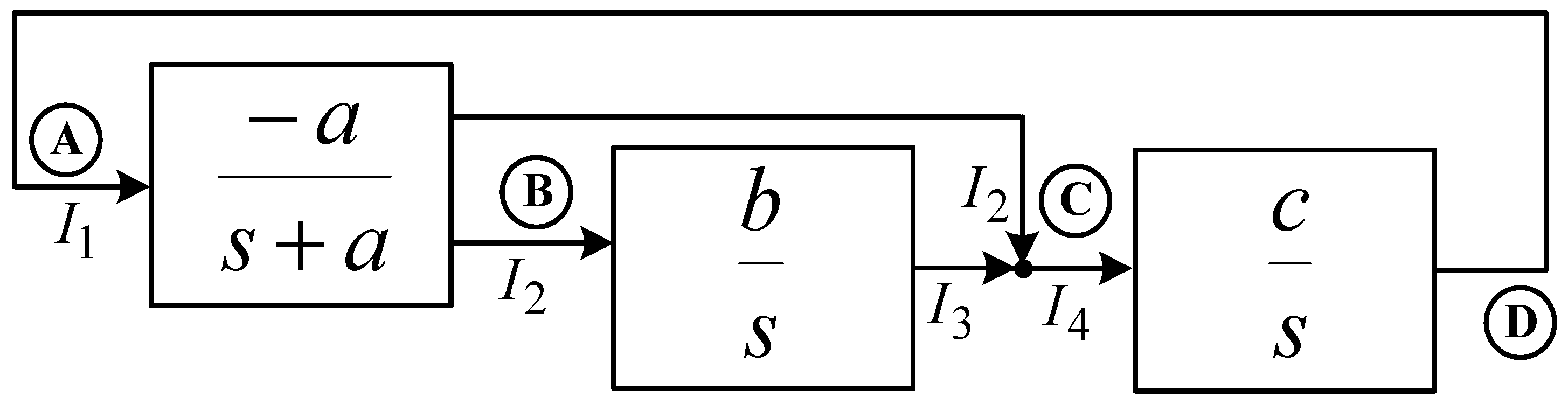Figure 2. MO-CCCCTA symbol.
Figure 2. MO-CCCCTA symbol.Figure 3. The proposed third-order quadrature sinusoidal oscillator.
Figure 3. The proposed third-order quadrature sinusoidal oscillator.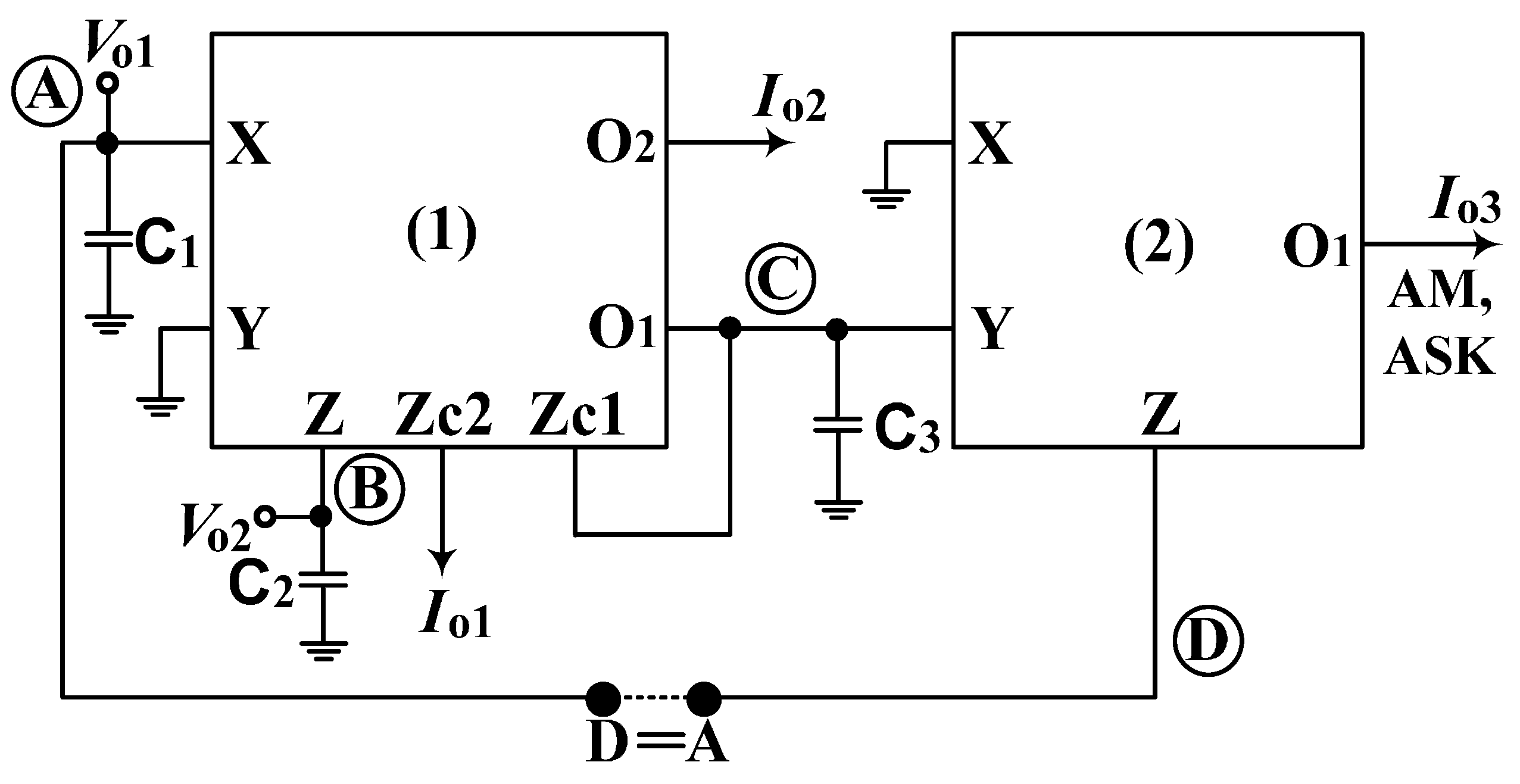Figure 4. Linear oscillatory system in Reference .
Figure 4. Linear oscillatory system in Reference .Figure 5. The internal construction of MO-CCCCTA.
Figure 5. The internal construction of MO-CCCCTA.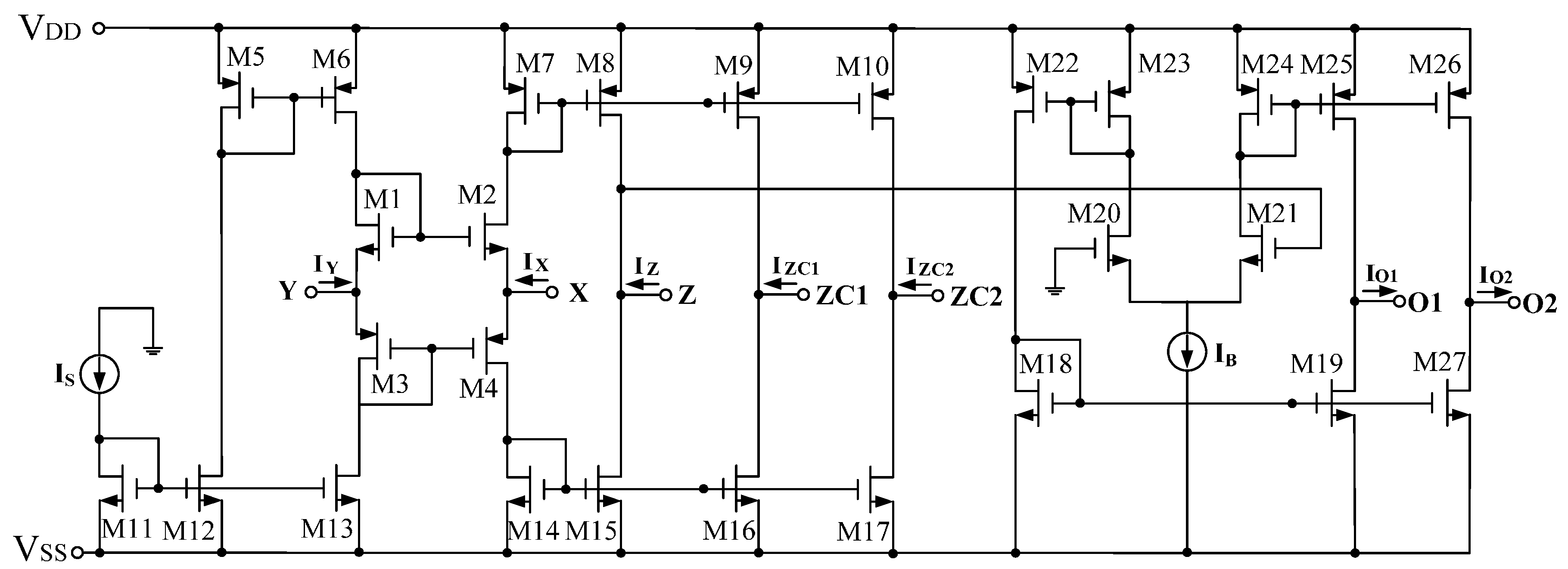Figure 6. Variation of transconductance with bias current.
Figure 6. Variation of transconductance with bias current.Figure 7. Simulated quadrature voltage outputs Vo1 (blue) and Vo2 (red).
Figure 7. Simulated quadrature voltage outputs Vo1 (blue) and Vo2 (red).Figure 8. Simulated quadrature current outputs Io1 (blue) and Io2 (red).
Figure 8. Simulated quadrature current outputs Io1 (blue) and Io2 (red).Figure 9. Simulated result of operation as an AM signal generator when IB2 is the applied sinusoidal signal.
Figure 9. Simulated result of operation as an AM signal generator when IB2 is the applied sinusoidal signal.Figure 10. Simulated result of operation as an AM signal generator when IB2 is the applied triangular signal.
Figure 10. Simulated result of operation as an AM signal generator when IB2 is the applied triangular signal.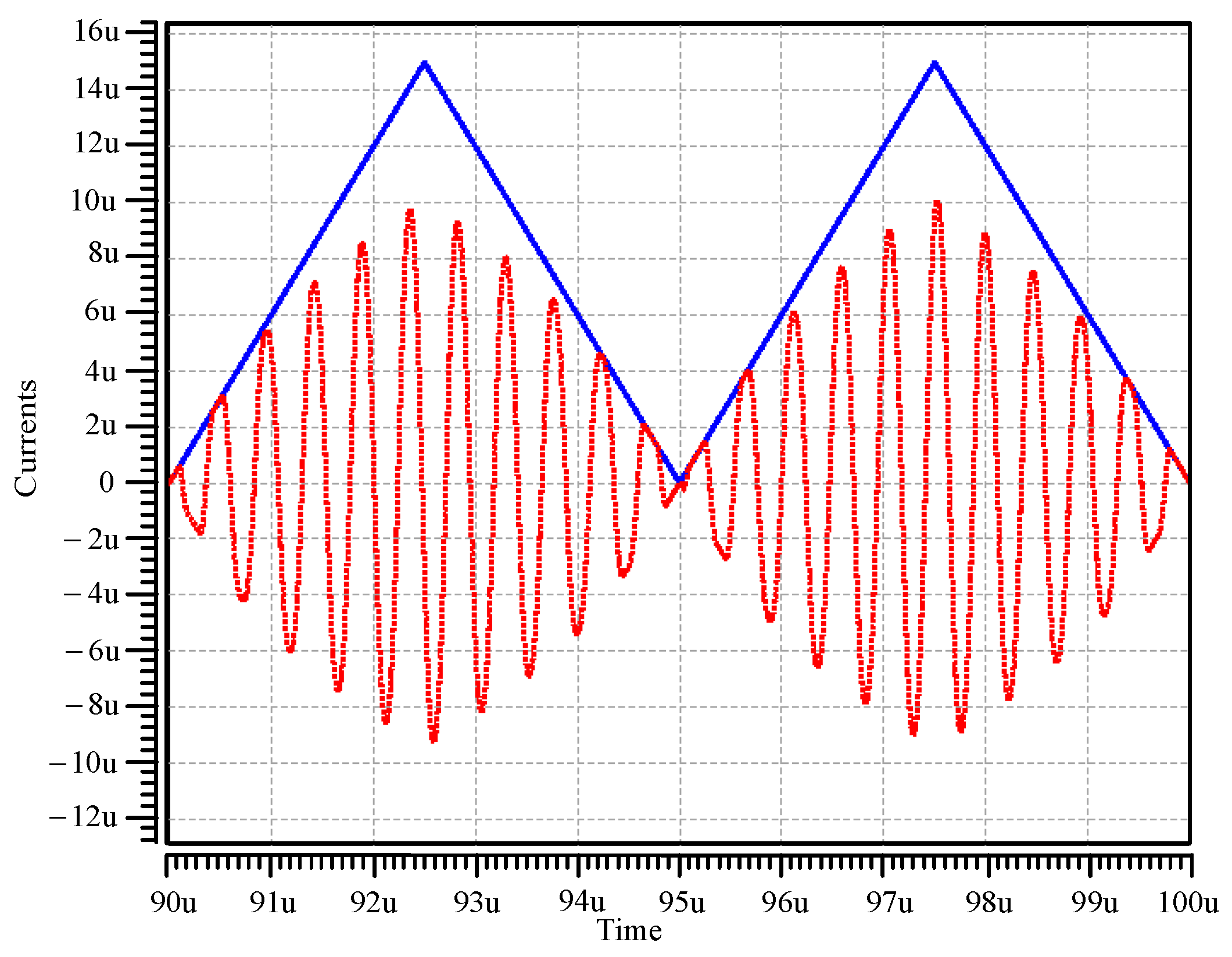Figure 11. Simulated result of operation as an ASK signal generator when IB2 is the applied pulse signal.
Figure 11. Simulated result of operation as an ASK signal generator when IB2 is the applied pulse signal.Figure 12. A possible implement method of the proposed oscillator by using commercially available ICs.
Figure 12. A possible implement method of the proposed oscillator by using commercially available ICs.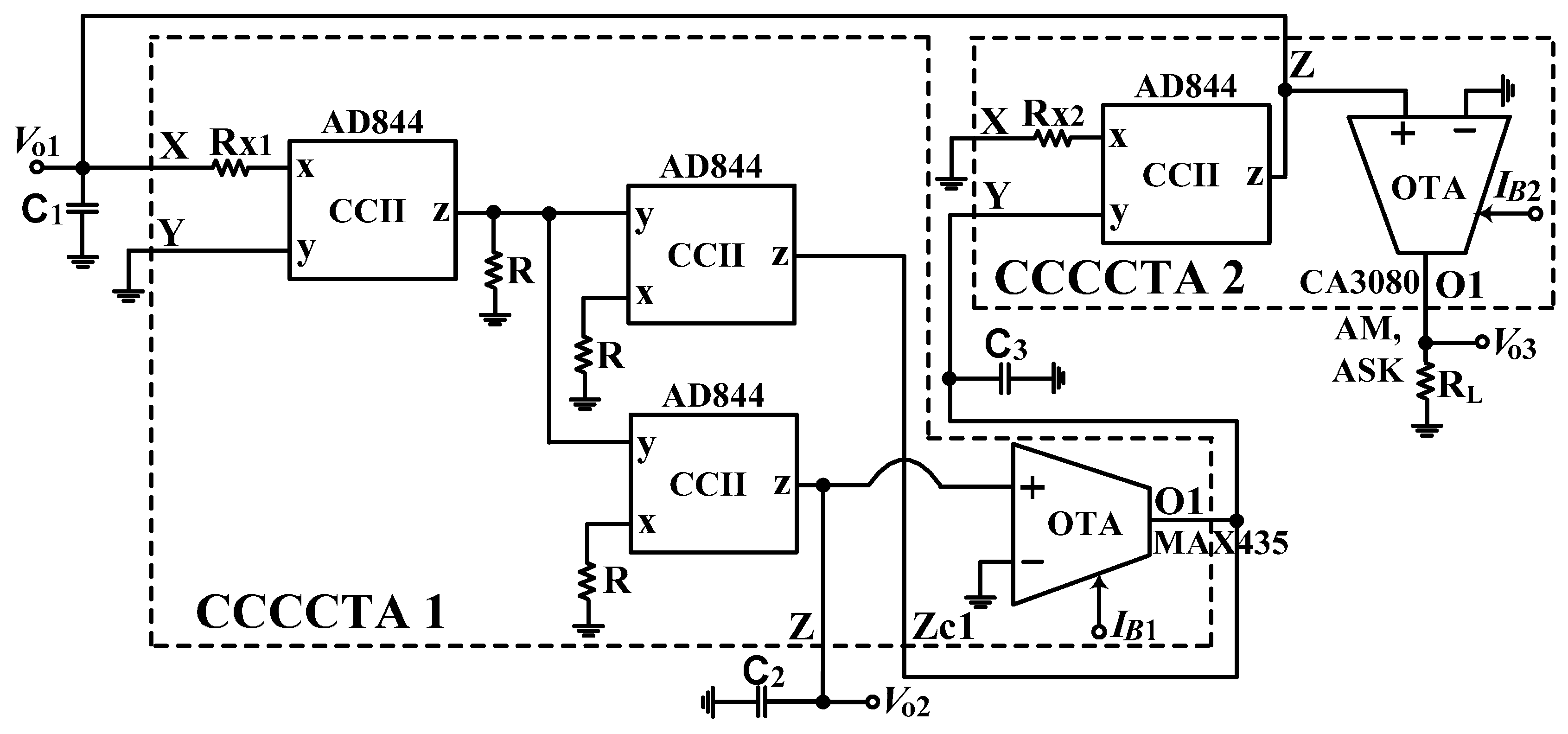Figure 13. Tested frequency range of AD844.
Figure 13. Tested frequency range of AD844.Figure 14. The experimental evidence frequency ranges of AD844 when R1 = R2 = 1 kΩ (yellow line; R1 = R2 = 5 kΩ (cyan line); and R1 = R2 = 10 kΩ (purple line).
Figure 14. The experimental evidence frequency ranges of AD844 when R1 = R2 = 1 kΩ (yellow line; R1 = R2 = 5 kΩ (cyan line); and R1 = R2 = 10 kΩ (purple line).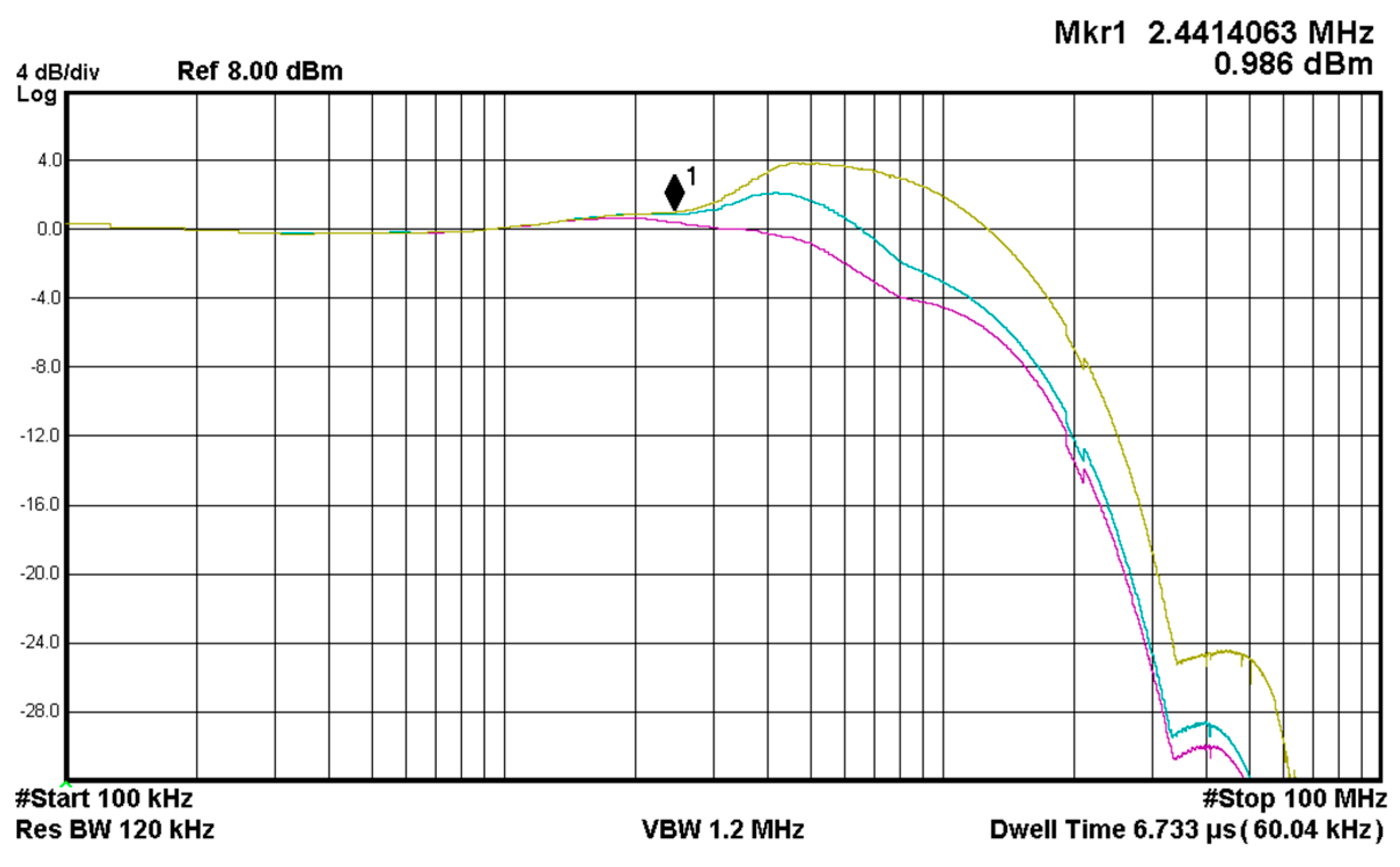Figure 15. The experimental waveforms of the quadrature outputs Vo1 (channel 1) and Vo2 (channel 2).
Figure 15. The experimental waveforms of the quadrature outputs Vo1 (channel 1) and Vo2 (channel 2).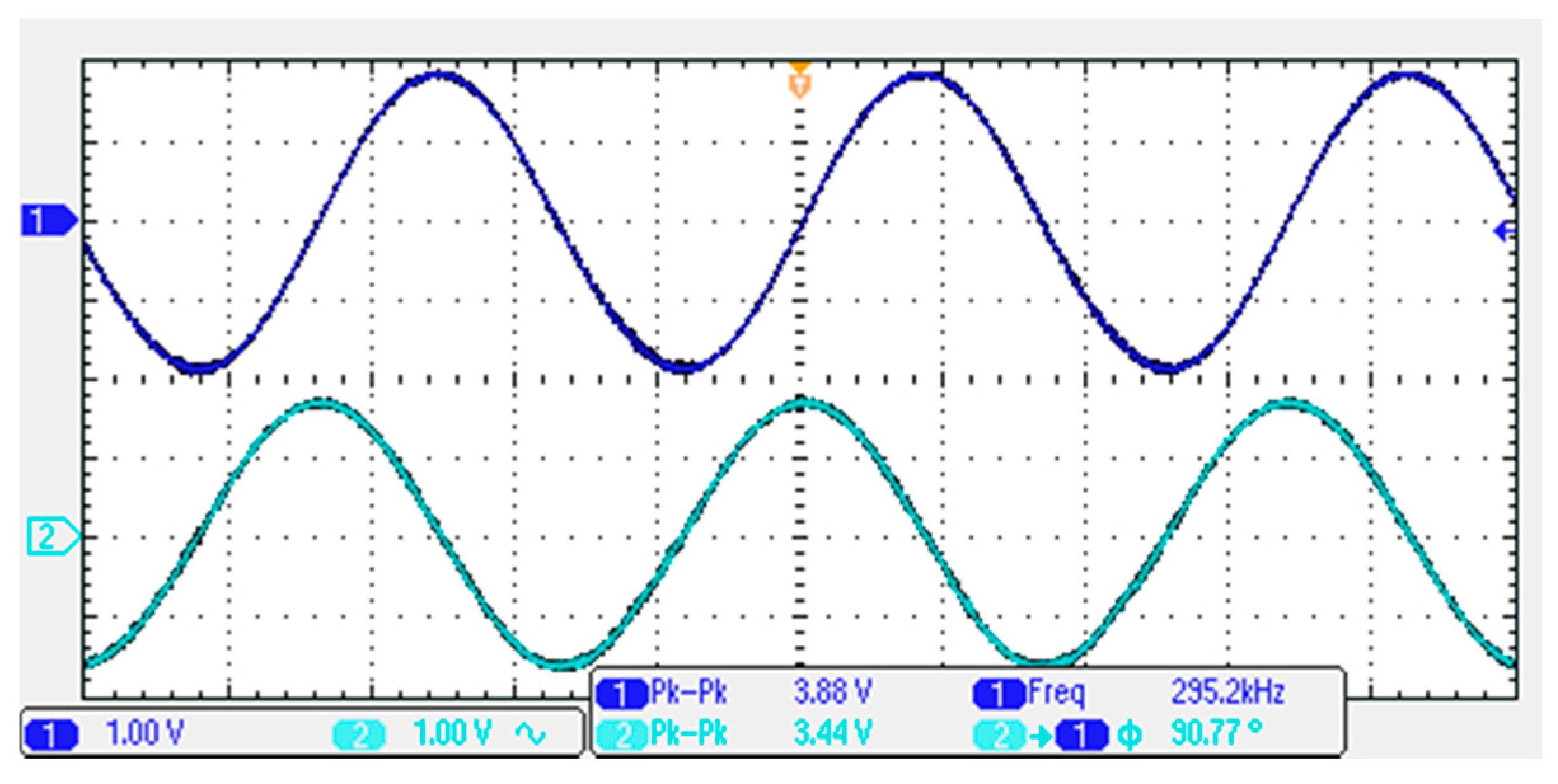Figure 16. The experimental result for XY plot of Vo1 and Vo2 outputs in Figure 15.
Figure 16. The experimental result for XY plot of Vo1 and Vo2 outputs in Figure 15.Figure 17. Measured result of operation as an AM signal generator (channel 2) when IB2 is the applied sinusoidal signal (channel 1).
Figure 17. Measured result of operation as an AM signal generator (channel 2) when IB2 is the applied sinusoidal signal (channel 1).Figure 18. Measured result of operation as an AM signal generator (channel 2) when IB2 is the applied triangular signal (channel 1).
Figure 18. Measured result of operation as an AM signal generator (channel 2) when IB2 is the applied triangular signal (channel 1).Figure 19. Measured result of operation as an AM signal generator (channel 2) when IB2 is the applied pulse signal (channel 1).
Figure 19. Measured result of operation as an AM signal generator (channel 2) when IB2 is the applied pulse signal (channel 1).Figure 20. The experimental frequency spectrum of Vo1.
Figure 20. The experimental frequency spectrum of Vo1.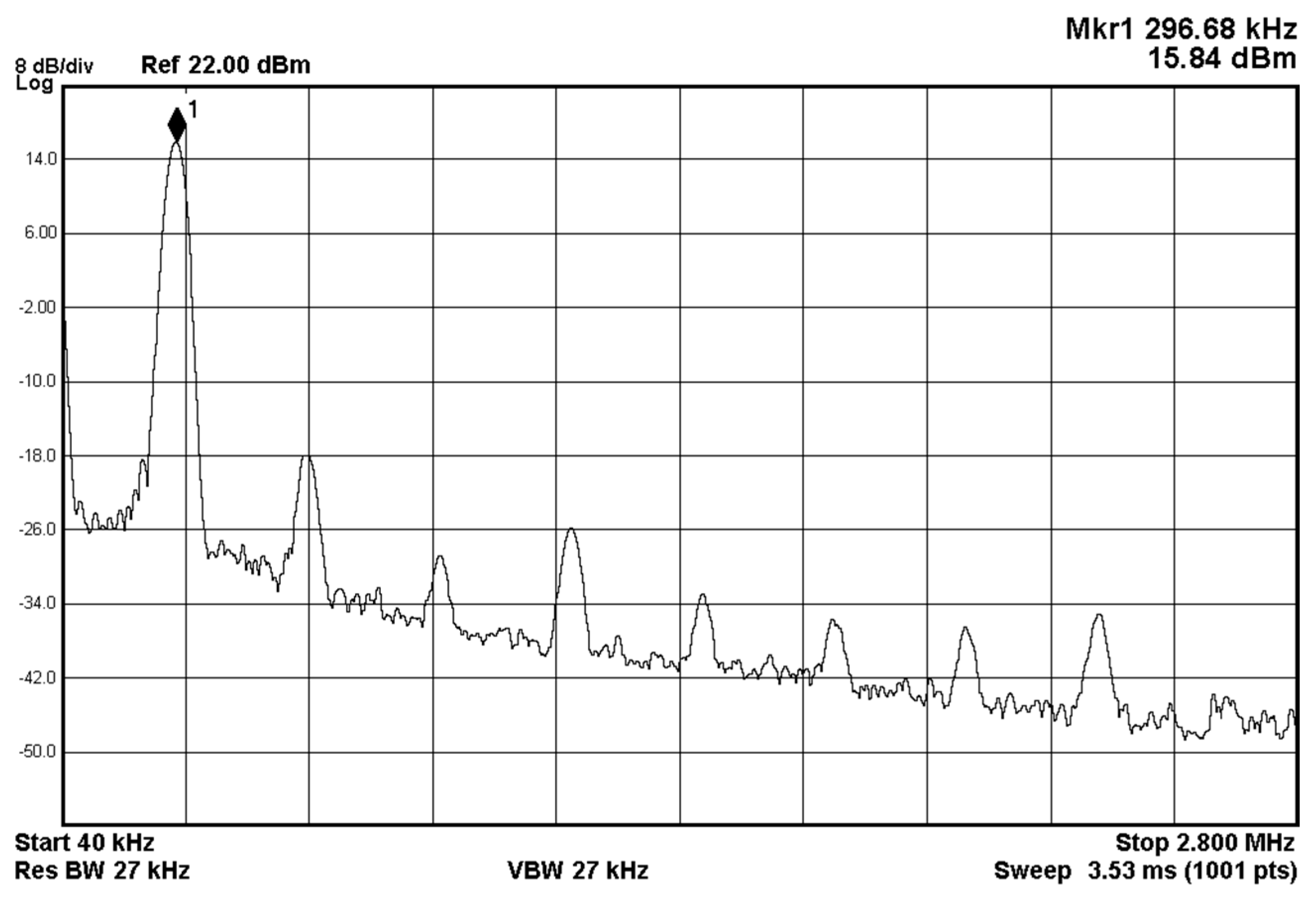Figure 21. The measured phase noise of the proposed oscillator of Vo1.
Figure 21. The measured phase noise of the proposed oscillator of Vo1.Figure 22. The measured RMS jitter of the proposed oscillator at an operating frequency of 295.2 kHz.
Figure 22. The measured RMS jitter of the proposed oscillator at an operating frequency of 295.2 kHz.Table 1. Number of component and number of output signal comparisons with previous third-order oscillators.
Table 1. Number of component and number of output signal comparisons with previous third-order oscillators.
ReferenceNo. of Components UsedNo. of Output Signals Used
Active ElementsC/R/Resistorless StructureVoltageCurrent
 Figure 73 OTAs3/0/yes10
4 MO-CCCIIs3/0/yes04
 Figure 13 CCIIs3/5/no20
2 MO-CCCCTAs3/0/yes02
 Figure 23 MO-DVCCs3/3/no52
 Figure 33 VC-DVCCs3/0/yes32
3 MO-CCCIIs3/0/yes24
3 MO-CDTAs3/0/yes22
2 MO-CCIIs3/3/no22
3 MO-DVCCs3/3/no24
 Figure 33 OTRAs3/5/no20
 Figure 4a2 MO-DVCCTAs 3/1/no33
 Figure 4b2 MO-DVCCTAs 3/2/no33
This work2 MO-CCCCTAs3/0/yes23
Table 2. Characteristic comparisons with previous third-order oscillators.
Table 2. Characteristic comparisons with previous third-order oscillators.
ReferenceElectronic Tuning FO without Disturbing COIndependent Control of CO and FOControllable Amplitude of AM/ASK
 Figure 7nonono
yesyesno
 Figure 1nonono
yesyesno
 Figure 2noyesno
 Figure 3yesyesno
yesyesno
yesyesno
noyesno
nonono
 Figure 3noyesno
 Figure 4anoyesno
 Figure 4bnonono
This workyesyesyes
Table 3. The aspect ratios of the CMOS transistors in MO-CCCCTA implementation.
Table 3. The aspect ratios of the CMOS transistors in MO-CCCCTA implementation.
TransistorsL (µm)W (µm)
M1–M20.357
M3–M40.3514
M5–M100.520
M11–M17, M20, M210.510
M18, M19, M270.88
M22–M260.825
Table 4. Total harmonic distortion analysis of Vo1 in Figure 3.
Table 4. Total harmonic distortion analysis of Vo1 in Figure 3.
Harmonic NumberFrequency (MHz)Fourier ComponentPhase (degree)
12.122131.6570 m152.1558
24.2441.7963 m−145.6957
36.3661.3875 m−16.7744
48.488280.3543 u−35.0773
510.61059.7176 u−158.4953
612.732139.7939 u−8.9033
714.854117.2583 u−9.5498
816.976100.4933 u2.8933
919.098101.7488 u8.4091
DC component = 3.419 × 10−3
Total harmonic distortion = 1.7465%
Table 5. Total harmonic distortion analysis of Vo2 in Figure 3.
Table 5. Total harmonic distortion analysis of Vo2 in Figure 3.
Harmonic NumberFrequency (MHz)Fourier ComponentPhase (degree)
12.122131.7010 m117.7808
24.244557.7167 u107.6894
36.366591.5883 u51.9561
48.488195.7997 u27.5504
510.61071.6988 u2.9989
612.73264.9872 u16.6679
714.85467.4512 u7.9859
816.97660.2372 u11.2194
919.09851.7097 u15.5339
DC component = −4.142 × 10−4
Total harmonic distortion = 644.0952 m%
Table 6. Total harmonic distortion analysis of Io1 in Figure 3.
Table 6. Total harmonic distortion analysis of Io1 in Figure 3.
Harmonic NumberFrequency (MHz)Fourier ComponentPhase (degree)
12.12217.5810 u−27.9532
24.244175.7963 n−143.2494
36.366204.1361 n155.1108
48.48857.0393 n145.0789
510.61012.1911 n−93.1991
612.73216.2208 n−141.3161
714.8546.6238 n157.6872
816.97610.0840 n161.5075
919.09813.2706 n−179.8498
DC component = −2.993 × 10−8
Total harmonic distortion = 1.5739%
Table 7. Total harmonic distortion analysis of Io2 in Figure 3.
Table 7. Total harmonic distortion analysis of Io2 in Figure 3.
Harmonic NumberFrequency (MHz)Fourier ComponentPhase (degree)
12.12217.2823 u−117.8809
24.24472.1321 n106.2341
36.366156.5673 n26.8057
48.48824.1643 n25.9664
510.6108.2084 n1.3462
612.7327.7736 n16.4662
714.8548.5334 n8.0360
816.9767.7471 n11.0721
919.0986.5087 n14.8607
DC component = −5.366 × 10−8
Total harmonic distortion = 1.0122%